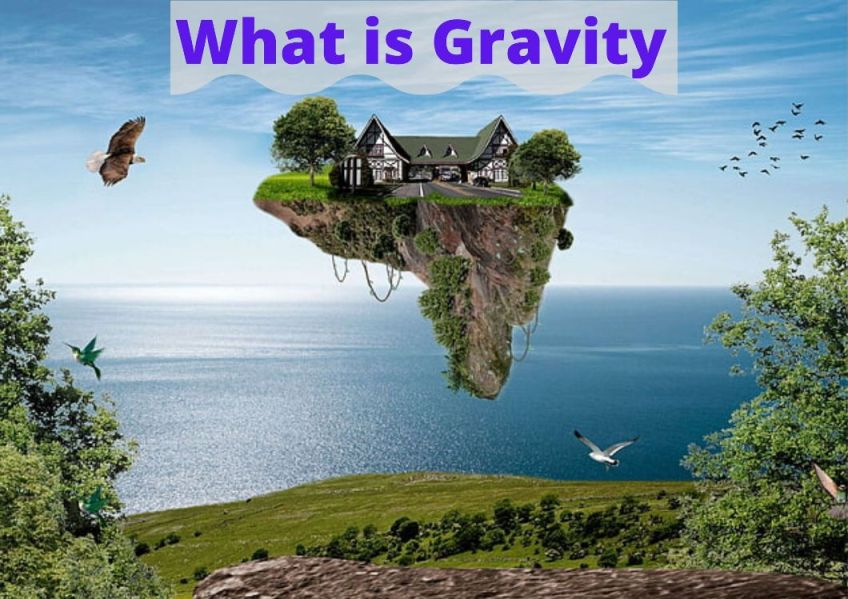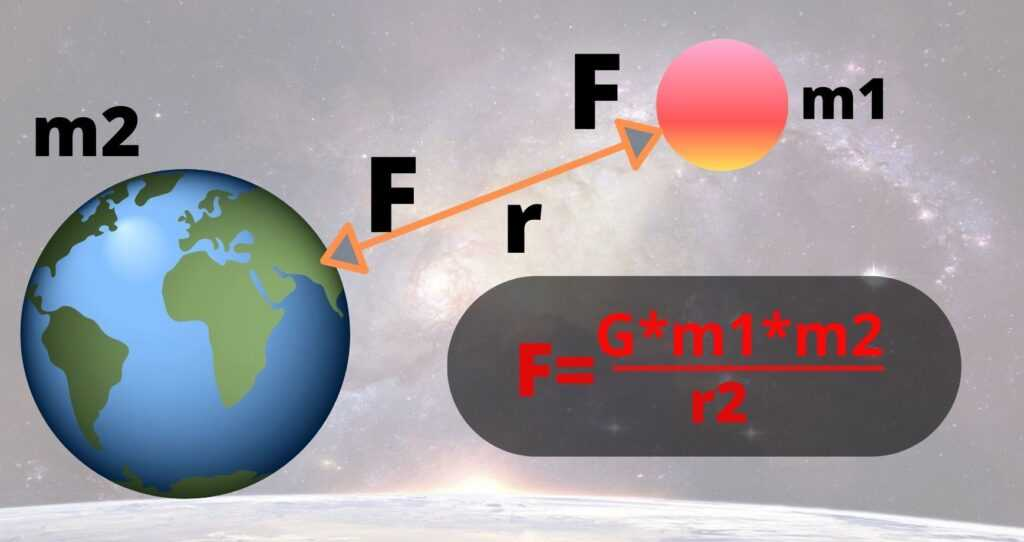# What is Gravity and Gravitational Force? Explanation!Gravity is the force of attraction between two objects which have a definite mass or energy. Due to gravity, two things get attracted to each other. These things may be small atoms, large objects like planets, stars, galaxies, etc.

Though, generally, the term Gravity and Gravitational Force are used interchangeably. But in a few specific types of studies, gravity and gravitation are used with different meanings. Gravitational force mainly focuses on two objects of field, while gravity talks about one specific object by which the force is applied.

## The general meaning of Gravity and Gravitational Force

The word gravity is taken from the Latin word ‘gravitas’, that’s meaning ‘weight’. Though gravity is the weakest fundamental force of nature, so it is almost negligible for atoms but it plays an important role when considering large and massive bodies.

(“When you throw any objects towards up why does it come down, why doesn’t it go upward for always…?” This is one of the most asked question in childhood by a child’s relatives…Doesn’t it…? So here’s the answer, it’s because of the gravity of the earth.)

The terms gravity and gravitational force both have different meanings but in general, we consider them the same. Here both are described in a simple meaning:-

• The attraction force between two objects is called gravitation force, these objects may be from anywhere in the universe.
• While in the case of gravity, it is the force of attraction of a body towards the center of the earth.
 Gravitational force is the broad term used for the universe while gravity force is considered only on earth.Gravity is the weakest fundamental force of nature. To define gravitation Scientists have described it many times and still working to understand its behavior. Here we have mentioned two main theories to get it properly:

1.  Newton’s law of gravitation,
2.  Gravity by Einstein’s general theory of relativity.

## Newton’s law of gravitation

In 1687 AD, Sir Isaac Newton gave a law which is also called the Universal law of gravitation and thus he first discovered gravity.

(“should I tell you about the story of an apple falling down…?” Ok leave it, I think your judgemental relatives or teacher has told you about it. -Lol)

The universal law of gravitation by Newton- “All bodies in the universe attract other bodies with a force that is directly proportional to their masses and inversely proportional to the square of the distance between them, and this force is called gravitational force.”

If we consider two bodies with their mass ‘m1‘ and ‘m2‘ and the distance between them is ‘r’, then we found two stats:

F ∝ m1 × m2

F ∝ 1/r2

⇒ F ∝ m1 × m2/r2

⇒ F=Gm1 × m2/r2

Here ‘G’ is a proportional constant also known as the universal gravitational constant.

The mathematical value of universal constant, G=6.67 × 10-11  Nm2/kg## Gravity by Einstein’s General Theory of Relativity

Though Newton’s law of gravity is applicable to the objects that move slowly compare to the speed of light. It gives the approximate value for the gravitational effect of any object. Whereas a theory given by Albert Einstein in 1915 describes gravity more accurately, and this theory is known as the “general theory of relativity”.

According to Einstein’s general theory of relativity, gravity is not a force, it is a space-time curvature caused by the mass of any object. This theory is mostly applicable to the gravitation of a body with high mass or density.

The mass of any object is the proportion of the space-time distortion. The objects with more mass distort the space-time more, whereas the low mass object has low distortion and low gravity.

Here below you will get some other terms related to gravity and gravitational force:

#### 1. Acceleration due to the gravity of Earth

The gravitational force which is considered on earth is called gravity. Acceleration due to gravity is the pulling acceleration of a body due to the gravitational force of the earth.

This acceleration due to gravity is represented by a small letter ‘g’ and its value on the surface of the earth is 9.8m/sec2.

If we consider a small object is placed outside of the earth at some height and another object is the earth itself, then the earth will start pulling this small object with acceleration due to gravity ‘g’.

#### 2. The relation between weight and gravity

The weight of an object is equal to the gravitational force of the object towards the earth’s center or the weight of an object is the force of gravity on the object if it is placed on the surface of the earth. So both the term weight and force of gravity are the same.

Case 1st- if the object is placed on the surface of the earth:

Weight = Force of Gravity

If a body of mass m is placed on the surface of earth then its weight, W=mg

and also the force of gravity, Fg=mg

where ‘g’ is the acceleration due to gravity.

Case 2nd- if the object is placed on the height of ‘h’ from the center of the earth:

Weight equals ≠ Force of Gravity

If a body of mass m is placed on any height from the earth’s surface, W=mg

And the force of gravity, Fg=G × Me × m/h2

Where ‘Me’  is the mass of the earth.

Weight is a variable term that depends on the value of acceleration due to gravity ‘g’.

#### 3. Weight on Other Planets

The weight of any object depends on the value of ‘g’. The value of ‘g’ also differs for each and every planet, whereas the mass of the body is always constant.

Here is the list of the value of ‘g’ on every planet with the respective weight considering the mass of any small body ‘m=10 kg’ which is placed on the surface of that planet. It means if someone’s mass on earth is 10 kg then the weight of him/her would be around 98 N (usually we call it 98 kg weight, instead of 98 N weight) on earth.

 Planets Value of ‘g’ in m/sec2 Weight (W=mg) in N Mercury 3.7 m/sec2 37 N Venus 8.87 m/sec2 88.7 N Earth 9.8 m/sec2 98 N Mars 3.71 m/sec2 37.1 N Jupiter 24.79 m/sec2 247.9 N Saturn 10.44 m/sec2 104.4 N Uranus 8.87 m/sec2 88.7 N Neptune 11.15 m/sec2 111.5 N
 Check about:- All 8 Planet Facts

The value of ‘g’ on the Moon is 1.62 m/secand the weight is 16.2 N if the mass of the body is 10 kg.

So, this was some important information about Gravity and Gravitational Force. Here you got to know some relevant theories about gravity. Hope you have got to know some important knowledge. Now you can check below some awesome articles.

Check the below articles:-

## 2 thoughts on “What is Gravity and Gravitational Force? Explanation!”

1.Hello! planetseducation.com site is very useful and inspirational, and I do not think I arrived without a reason.

1.That’s great. Good Luck.